Right Triangles
edHelper subscribers - Create a new printable

Number of Keys
 Select the number of different printables: 1 key 2 keys 3 keys 4 keys 5 keys

Number of Pages (for each key)
 Select the number of pages: 1 page 2 pages 3 pages 4 pages

Include an answer key (answer keys will be at the end of the printable)
High School Geometry
High School Geometry
Math

 Name _____________________________Date ___________________ (Key 1 - Answer ID # 0712029)
1.

Use the diagram below to answer the following questions.
(a) If m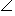K = 29, then mKLM = ______ and mJ = ______.
(b)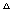LMK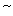____________
(c) JL is the geometric mean between ______ and ______.
(d) KL is the geometric mean between ______ and ______.
(e) LM is the geometric mean between ______ and ______.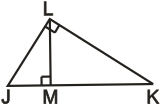2.

If AD = 3 and BD = 6, which could be possible?
(I) CD = 3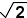(II) AC = 3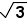(III) BC = 3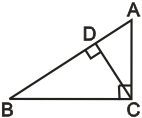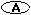I only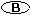I and II only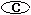I and III only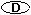I, II, and III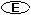None of the above

3.

InABC, AC = x - 8, BC = 5x - 144, and mC = 90. Find the value of x that makesABC a right isosceles triangle.

4.

In rightABC, altitude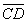is drawn to the hypotenuse. If AD = 8, BD = 18, and CD = x + 9, find the length of the altitude.

5.

Find the length of the diagonal of a rectangle with sides 12 cm and 12cm.

6.

The diagonal of a square box top is 14 feet. Find the length of a side of the box top.

7.

A rectangle has a diagonal of 12 and length of 4. Find the area of the rectangle.

8.

Find the length of the altitude to the base of an isosceles triangle with sides 15, 15, and 24.

9.

Find the length of a diagonal of a square with perimeter of 8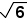.

10.

InABC, mA = 30and mC = 90. If BC is 13 less than AB, find the length of the hypotenuse.

Right Triangles
edHelper subscribers - Create a new printable

Number of Keys
 Select the number of different printables: 1 key 2 keys 3 keys 4 keys 5 keys

Number of Pages (for each key)
 Select the number of pages: 1 page 2 pages 3 pages 4 pages